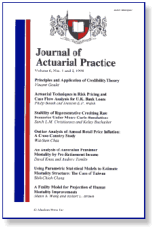## Finance Department## Journal of Actuarial Practice 1993-2006

2001

Article

#### Citation

Journal of Actuarial Practice 9 (2001), pp. 131-150

Copyright 2001 Absalom Press

#### Abstract

In this paper we consider linear empirical Bayes estimation of survival probabilities with partial data from right-censored and possibly left-truncated observations. Such data are produced by studies in which the exact times of death are not recorded and the length of time that each subject may be under observation cannot exceed one unit of time. We obtain asymptotically optimal linear empirical Bayes estimators, with respect to the squared error loss function, under the assumption that the probability of death under observation in a unit time interval is proportional to the length of observation. This assumption is sometimes implied by Balducci's assumption and sometimes is implied by the assumption of uniform distribution of deaths.

COinS